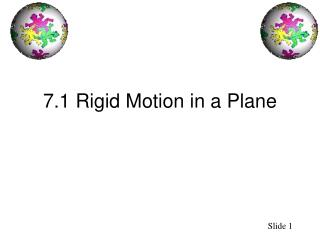DownloadDownload Presentation7.1 Rigid Motion in a Plane

# 7.1 Rigid Motion in a Plane

Download Presentation## 7.1 Rigid Motion in a Plane

- - - - - - - - - - - - - - - - - - - - - - - - - - - E N D - - - - - - - - - - - - - - - - - - - - - - - - - - -
##### Presentation Transcript

1. 7.1 Rigid Motion in a Plane

2. Objectives/Assignment • Identify the three basic rigid transformations • Assignment: 2-38 even

3. Identifying Transformations • Figures in a plane can be • Reflected • Rotated • Translated • To produce new figures. The new figures is called the IMAGE. • The original figures is called the PREIMAGE. • The operation that MAPS, or moves the preimage onto the image is called a TRANSFORMATION.

4. What will you learn? • Three basic transformations: • Reflections • Rotations • Translations • And combinations of the three. • For each of the three transformations on the next slide, the blue figure is the preimage and the red figure is the image. We will use this color convention throughout the rest of the book.

5. Copy this down Rotation about a point Reflection in a line Translation

6. Some facts • Some transformations involve labels. • When you name an image, take the corresponding point of the preimage and add a prime symbol. • For instance, if the preimage is A, then the image is A’, read as “A prime.”

7. Use the graph of the transformation at the right. Name and describe the transformation. Name the coordinates of the vertices of the image. Is ∆ABC congruent to its image? Example 1: Naming transformations

8. Name and describe the transformation. The transformation is a reflection in the y-axis. You can imagine that the image was obtained by flipping ∆ABC over the y-axis. Example 1: Naming transformations

9. Name the coordinates of the vertices of the image. The cordinates of the vertices of the image, ∆A’B’C’, are A’(4,1), B’(3,5), and C’(1,1). Example 1: Naming transformations

10. Is ∆ABC congruent to its image? Yes ∆ABC is congruent to its image ∆A’B’C’. One way to show this would be to use the DISTANCE FORMULA to find the lengths of the sides of both triangles. Then use the SSS Congruence Postulate Example 1: Naming transformations

11. ISOMETRY • An ISOMETRY is a transformation the preserves lengths. • Isometries also preserve angle measures, parallel lines, and distances between points. • Transformations that are isometries are called RIGID TRANSFORMATIONS.

12. Which of the following appear to be isometries? This transformation appears to be an isometry. The blue parallelogram is reflected in a line to produce a congruent red parallelogram. Ex. 2: Identifying Isometries

13. Which of the following appear to be isometries? This transformation is not an ISOMETRY because the image is not congruent to the preimage Ex. 2: Identifying Isometries

14. Which of the following appear to be isometries? This transformation appears to be an isometry. The blue parallelogram is rotated about a point to produce a congruent red parallelogram. Ex. 2: Identifying Isometries

15. You can describe the transformation in the diagram by writing “∆ABC is mapped onto ∆DEF.” You can also use arrow notation as follows: ∆ABC  ∆DEF The order in which the vertices are listed specifies the correspondence. Either of the descriptions implies that A  D, B  E, and C  F. Mappings

16. In the diagram ∆PQR is mapped onto ∆XYZ. The mapping is a rotation. Given that ∆PQR  ∆XYZ is an isometry, find the length of XY and the measure of Z. Ex. 3: Preserving Length and Angle Measures 35°

17. SOLUTION: The statement “∆PQR is mapped onto ∆XYZ” implies that P  X, Q  Y, and R  Z. Because the transformation is an isometry, the two triangles are congruent. So, XY = PQ = 3 and mZ = mR = 35°. Ex. 3: Preserving Length and Angle Measures 35°

18. Using Transformations in Real Life • Look at sample problems on page 398.